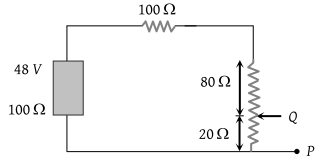NEET Questions Solved

In the circuit, the potential difference across PQ will be nearest to(1) 9.6 V

(2) 6.6 V

(3) 4.8 V

(4) 3.2 V

(4) Potential difference across PQ i.e. p.d. across the resistance of 20Ω, which is V = i × 20

and $i=\frac{48}{\left(100+100+80+20\right)}=0.16A$

$V=0.16×20=3.2V$

Difficulty Level:

• 11%
• 24%
• 26%
• 40%